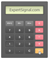DeMarker Indicator

Usage: Analyzing Market Trends / Determining Timing

◙ Trading: Evaluating Trends and Spotting Potential Price Exhaustion

Standard Settings: 14 PeriodsIntroduction to the DeMarker Indicator

The indicator was developed by Tom Demarker, founder, and CEO of the DeMark Analytics. DeMarker indicator has the ability to evaluate areas of likely price trend formation or price exhaustion, prior to their occurrence.

-The DeMarker focuses on market direction but also on the potential of perfect timingCalculating DeMarker Indicator

The DeMarker indicator is the sum of all price increment values recorded during the "i" period divided by the price minima:

■ The DeMax (i) is calculated:

If high (i) > high (i-1), then DeMax (i) = high (i) – high (i-1),

otherwise DeMax (i) = 0

■ The DeMin (i) is calculated:

If low (i) < low (i-1), then DeMin (i) = low (i-1) – low (i),

otherwise DeMin (i) = 0

■ DeMarker value is calculated as:

DMark (i) = SMA ( DeMax, T ) / ( SMA ( DeMax, T ) + SMA ( DeMin, T ) )

Where:

T= the number of periods

SMA = Simple Moving AverageTrading with the DeMarker Indicator

The indicator can help traders to evaluate the current phase of the trend and a potential exhaustion point:

• Evaluate market trends
• Spot points of potential price exhaustionPlatform Setup

□ GO TO → INDICATORS → OSCILLATORS → DEMARKER OSCILLATOR

□ SETTINGS → 14 PERIODS

DeMarker Indicator